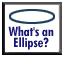# Kepler's LawsJohannes Kepler (1571-1630) challenged the belief that planets moved in perfectly circular orbits. Using data collected by the Danish astronomer Tycho Brahe, Kepler determined that the paths of the planets were ellipses, and formulated his three laws to explain and predict planetary motion.

FIRST LAW: Each planetary orbit is an ellipse, with the Sun at one focus of the ellipse.

SECOND LAW: The line joining the planet to the sun sweeps out equal areas for equal time intervals. This means: The closer a planet is to the Sun in its orbit, the faster it moves.

THIRD LAW: The cube of a planet's semi-major axis of its orbit, divided by the square of its orbital period, is a constant. So, where d is the length of the semi-major axis and t is the time it takes for an orbit, d3 /t2 is the same for all planets. Simply put: The farther a planet is from the Sun, the longer it takes to complete an orbit.These laws are important for calculating the orbit of a planet with only partial information. In essence, the discovery of Neptune and Pluto involved a reverse application of these laws and the law of gravity, to predict where a planet might be based on how it was affecting the orbit of another planet.

Back to Newton's Laws